Publication date: 04/12/2021

## Tests That the Variances Are Equal

#### F Ratio

O’Brien’s test constructs a dependent variable so that the group means of the new variable equal the group sample variances of the original response. The O’Brien variable is computed as follows: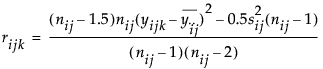where n represents the number of yijk observations.

Brown-Forsythe is the model F statistic from an ANOVA on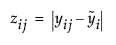where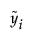is the median response for the ith level.

The Levene F is the model F statistic from an ANOVA on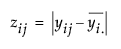where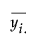is the mean response for the ith level.

Bartlett’s test is calculated as follows: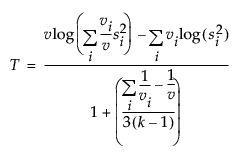where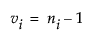and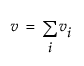and ni is the count on the ith level and si2 is the response sample variance on the ith level. The Bartlett statistic has a χ2-distribution. Dividing the Chi-square test statistic by the degrees of freedom results in the reported F value.

#### Welch’s Test F Ratio

The Welch’s Test F Ratio is computed as follows: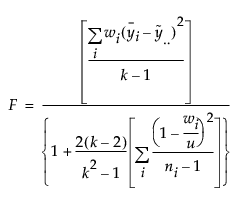where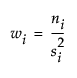,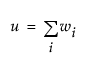,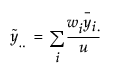,

and ni is the count on the ith level,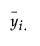is the mean response for the ith level, and si2 is the response sample variance for the ith level.

#### Welch’s Test DF Den

The Welch approximation for the denominator degrees of freedom is defined as follows: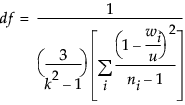where wi, ni, and u are defined as in the F ratio formula.

Want more information? Have questions? Get answers in the JMP User Community (community.jmp.com).